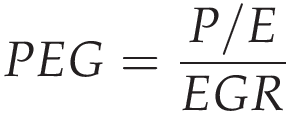# PEG Ratio Calculator

\$
\$
%
%

## Find the best stock opportunities with Calcopolis.

Our PEG ratio calculator helps you pick the stocks with the best growth potential by comparing the current company's performance to its growth rate.

In this article, we will discuss how to calculate the PEG ratio and how to interpret the returned values.

Finally, we will analyze how price-to-earnings growth affects a company's valuation.

## What is the PEG ratio?

A PEG ratio is a financial indicator of a company's growth potential. It analyzes the company's stock price, earnings, and growth potential.

PEG analysis extends the simple P/E ratio by considering the company's growth rate, which makes it especially useful in the case of growth stocks.

PEG ratio is widely used among experienced stock investors due to its simplicity and the amount of information it carries.

## PEG ratio formula

There are two formulas for calculating the stock PEG ratio.

The first is derived simply from the definition, and its P/E ratio is divided by the company's growth rate.Where:

• P/E - Price to earnings ratio
• EGR - Earnings growth rate

The second equation is a bit more complex:Where:

• P/E - Price to earnings ratio
• Retention - the retention rate of your customers
• ROE - Return on equity, more details visit our ROE calculator

## How to calculate the PEG ratio?

In order to illustrate the process of calculating the PEG ratio, let's analyze an example of an imaginary company ACME Corp.

The company has emitted 10 million shares, and its total market value is 100 million dollars.

The price per share equals:

Price per share = \$100,000,000 / \$10,000,000 = \$10

The company made \$10 million in profit in the last fiscal year, so earnings per share equal:

Earnings per share = Total earnings / Total number of shares = \$10,000,000 / 10,000,000 = \$1

In this case, the P/E ratio of this company looks as follows:

P/E = Price per share / Earnings per share = \$10 / \$1 = 10

The company works in a subscription model and has a retention rate of 80%.

The Return on equity (ROE) for the last fiscal year equals 10%.

Now we should put the data into the PEG formula.

PEG = (Price per share/ Earnings per share)/(Retention rate*Earnings growth) = (10 / 1) / 8 = 1.25

Note that a PEG ratio of 1.25 is noticeably lower than the company's P/E ratio.

## What is a good PEG ratio?

In theory PEG ratio of 1.0 is considered a balanced correlation between the stock price and the company's earnings.

Values lower than 1 may be considered an investment occasion. Whereas values above 1 are considered overpriced.

## Pros and cons of PEG ratio.

Pros. PEG ratio takes into account the company's growth rate.

This is the most significant advantage against a simple P/E comparison. Since the P/E indicator compares price to earnings, it tells nothing about the company's growth and potential.

Rapidly growing companies get noticed by advanced investors, and their price usually reflects that growth. If you analyze just P/E alone, you will miss many good opportunities.

Con. The PEG ratio says nothing about investment risk.

You should never forget that the PEG ratio is just a financial metric, and it tells nothing about the risk assigned to the investment in this company.

### Authors

Created by Lucas Krysiak on 2022-09-17 13:45:54 | Last review by Mike Kozminsky on 2022-09-17 16:14:23